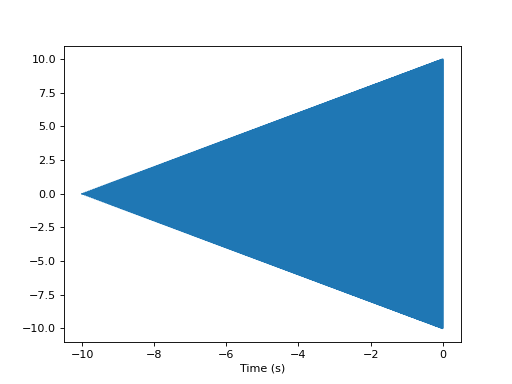# Making new waveform approximants available to PyCBC¶

## Adding a custom waveform model within a script¶

By example, the following script shows how to write a waveform model in the form required for PyCBC. We can also make this new waveform directly accessible by using the add_custom_waveform() function. If you are developing in a notebook or self-contained script, this may be what you want to do. However, if you want to make your waveform available to pycbc-based executables such as PyCBC Inference, also read the next section.

There are two kinds of models you can make. In the example below, we make a time-domain model. You can also make a freuqency-domain model. The only difference is that your function should return an instance of FrequencySeries and the required sample step option is delta_f instead of delta_t.

Each waveform generation function must take only keyword arguments, and should be able to take an arbitrary number of them. You may add new parameters as you like. These will be automatically useable by PyCBC Inference and other pycbc codes.

Each waveform model must have an associate approximant name, which identifies the model and distinguishes it from any other. If the name has already been used, you should select a different name. By default, an error will be raised unless overridden.

def test_waveform(**args):
import numpy
from pycbc.types import TimeSeries

flow = args['f_lower'] # Required parameter
dt = args['delta_t']   # Required parameter
fpeak = args['fpeak']  # A new parameter for my model

t = numpy.arange(0, 10, dt)
f = t/t.max() * (fpeak - flow) + flow
a = t

wf = numpy.exp(2.0j * numpy.pi * f * t) * a

# Return product should be a pycbc time series in this case for
# each GW polarization
#
#
# Note that by convention, the time at 0 is a fiducial reference.
# For CBC waveforms, this would be set to where the merger occurs
offset = - len(t) * dt
wf = TimeSeries(wf, delta_t=dt, epoch=offset)
return wf.real(), wf.imag()

import pylab
import pycbc.waveform

# This tells pycbc about our new waveform so we can call it from standard
# pycbc functions. If this were a frequency-domain model, select 'frequency'
# instead of 'time' to this function call.

# Let's plot what our new waveform looks like
hp, hc = pycbc.waveform.get_td_waveform(approximant="test",
f_lower=20, fpeak=50,
delta_t=1.0/4096)
pylab.figure(0)
pylab.plot(hp.sample_times, hp)
pylab.xlabel('Time (s)')

pylab.figure(1)
hf = hp.to_frequencyseries()
pylab.plot(hf.sample_frequencies, hf.real())
pylab.xlabel('Frequency (Hz)')
pylab.xscale('log')
pylab.xlim(20, 100)
pylab.show()(png, hires.png, pdf)(png, hires.png, pdf)

## Creating a plugin for PyCBC¶

To make a waveform model universally available to PyCBC so it can be called from PyCBC Inference, or the pycbc-based searched codes, you can create a plugin package which advertises your model. PyCBC will automatically detect your package and make your waveform model available for use.

The steps are:

• Create a waveform model just like as in the above example
• Create a python package for your module
• In your packages setup.py advertise that it contains a PyCBC compatible waveform model in it’s entry_points option.

Your setup.py should look like the following, the key addition being the entry_points parameter passed to setup.py.

setup (
name = 'pycbc-revchirp',
version = VERSION,
description = 'An example waveform plugin for PyCBC',
author = 'The PyCBC team',
author_email = 'alex.nitz@gmail.org',
url = 'http://www.pycbc.org/',
keywords = ['pycbc', 'signal processing', 'gravitational waves'],
install_requires = ['pycbc'],
py_modules = ['revchirp'],
entry_points = {"pycbc.waveform.td":"revchirp = revchirp:reverse_chirp_td",
"pycbc.waveform.fd":"revchirp = revchirp:reverse_chirp_fd"},
classifiers=[
'Programming Language :: Python',
'Programming Language :: Python :: 2.7',
'Programming Language :: Python :: 3.6',
'Intended Audience :: Science/Research',
'Natural Language :: English',
'Topic :: Scientific/Engineering',
'Topic :: Scientific/Engineering :: Astronomy',
'Topic :: Scientific/Engineering :: Physics',